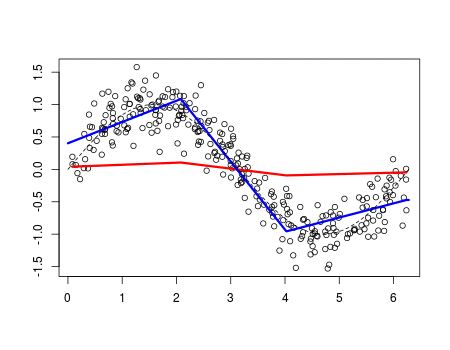# An Update on Boosting with Splines

July 2, 2015
By

Want to share your content on R-bloggers? click here if you have a blog, or here if you don't.

In my previous post, An Attempt to Understand Boosting Algorithm(s), I was puzzled by the boosting convergence when I was using some spline functions (more specifically linear by parts and continuous regression functions). I was using

```> library(splines)
> fit=lm(y~bs(x,degree=1,df=3),data=df)```

The problem with that spline function is that knots seem to be fixed. The iterative boosting algorithm is

• compute the residuals, including some shrinkage parameter,

then the strategy is to model those residuals

• at step , consider regression
• update the residuals

and to loop. Then set

I thought that boosting would work well if at step , it was possible to change the knots. But the outputwas quite disappointing: boosting does not improve the prediction here. And it looks like knots don’t change. Actually, if we select the ‘best‘ knots, the output is much better. The dataset is still

```> n=300
> set.seed(1)
> u=sort(runif(n)*2*pi)
> y=sin(u)+rnorm(n)/4
> df=data.frame(x=u,y=y)```

For an optimal choice of knot locations, we can use

```> library(freeknotsplines)
> xy.freekt=freelsgen(df\$x, df\$y, degree = 1,
+ numknot = 2, 555)```

The code of the previous post can simply be updated

```> v=.05
> library(splines)
> xy.freekt=freelsgen(df\$x, df\$y, degree = 1,
+ numknot = 2, 555)
> fit=lm(y~bs(x,degree=1,knots=
+ [email protected]),data=df)
> yp=predict(fit,newdata=df)
> df\$yr=df\$y - v*yp
> YP=v*yp
>  for(t in 1:200){
+    xy.freekt=freelsgen(df\$x, df\$yr, degree = 1,
+    numknot = 2, 555)
+ fit=lm(yr~bs(x,degree=1,knots=
+     [email protected]),data=df)
+    yp=predict(fit,newdata=df)
+    df\$yr=df\$yr - v*yp
+    YP=cbind(YP,v*yp)
+  }
>  nd=data.frame(x=seq(0,2*pi,by=.01))
>  viz=function(M){
+    if(M==1)  y=YP[,1]
+    if(M>1)   y=apply(YP[,1:M],1,sum)
+    plot(df\$x,df\$y,ylab="",xlab="")
+    lines(df\$x,y,type="l",col="red",lwd=3)
+    fit=lm(y~bs(x,degree=1,df=3),data=df)
+    yp=predict(fit,newdata=nd)
+    lines(nd\$x,yp,type="l",col="blue",lwd=3)
+    lines(nd\$x,sin(nd\$x),lty=2)}

>  viz(100)```I like that graph. I had the intuition that using (simple) splines would be possible, and indeed, we get a very smooth prediction.

R-bloggers.com offers daily e-mail updates about R news and tutorials about learning R and many other topics. Click here if you're looking to post or find an R/data-science job.
Want to share your content on R-bloggers? click here if you have a blog, or here if you don't.